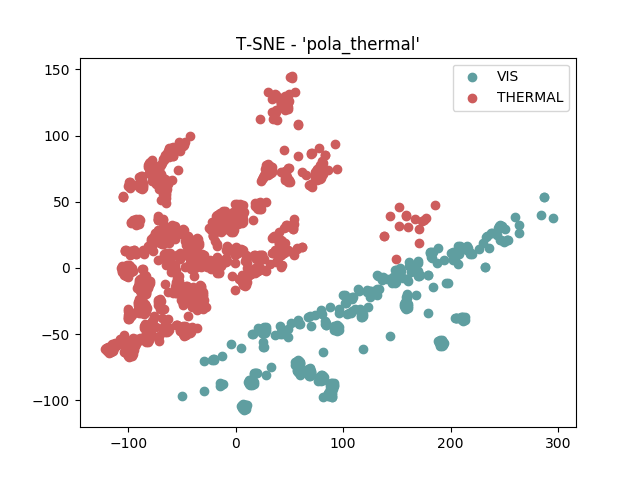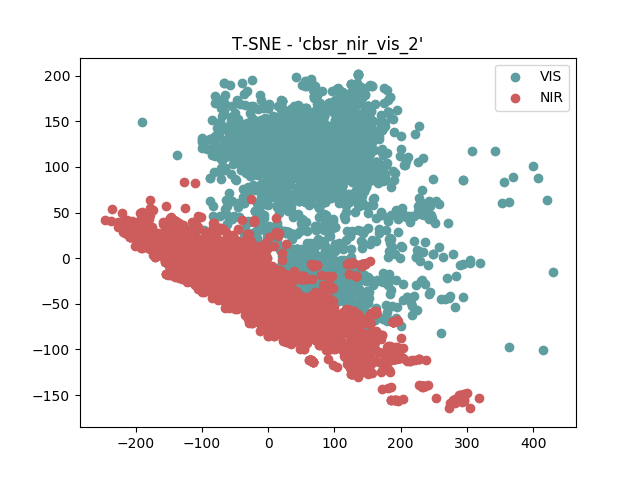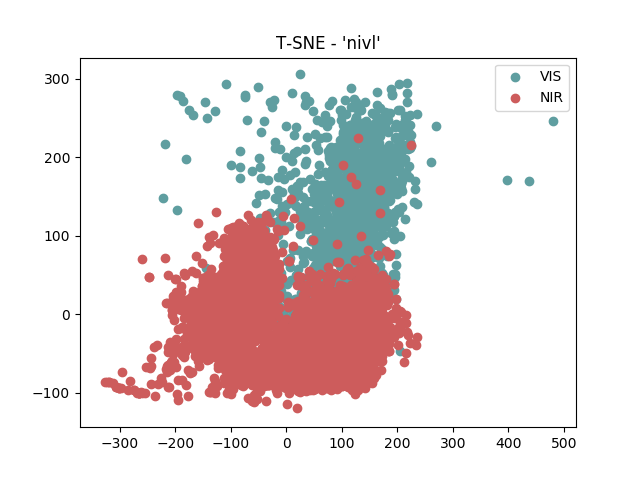# Transfer Learning¶

In this section we hypothesize that a shared latent subspace exists between two image modalities where the agreement is maximized. Given a set of covariates of two modalities $$x_A$$ and $$x_B$$, the goal is to find a common subspace $$\phi$$ where an arbitrary distance function $$d$$ can be applied It is expected that the distance is minimal when $$x_A$$ and $$x_B$$ belongs to the same client and maximal otherwise.

## First insights¶

Before any hypothesis on how to find this $$\phi$$, we have done two different analysis. The first analysis is exploratory and it consists on the observation of the distribution of the covariates $$x_A$$ and $$x_B$$ for different image modalities. To support this analysis we use the a well know algorithm that supports the visualization of high dimentional data called t-SNE. This algorithm converts similarities between data points to joint probabilities and minimizes the Kullback-Leibler divergence between the joint probabilities of the low-dimensional embedding and the high-dimensional data. Such exploratory analisys is carried out by a direct plot using the pixel space ($$x_A$$ and $$x_B$$).

The second analisys is more practical and it consists in the analisys of error rates in a closed-set scenario. The base question here at this point is to know if state-of-the-art face recognition systems can natually handle signals from heterogenous sources. We will explore six different DCNN models based on three base architectures for HFR. This set of experiments establishes the baseline results for further analysis.

The first is the VGG16-Face network REF[Parkhi2015], which was made publicly available by the Visual Geometry Group and consists of 16 hidden layers where the first 13 are composed by convolutions and pooling layers. The last three layers are fully-connected (named fc6, fc7, and fc8). As a feature representation, we use the embeddings produced by the ‘fc7’ layer. The input signal of such network are RGB images of $$224 \times 224$$ pixels. Since all our databases are one channel only (NIR, Sketch and Thermal), we convert them from one channel images to three channels by replicating the signal along the extra channels.

The second network used is the Light CNN. Xiang et al. in REF[WuXiang2015] proposed an architecture that has ten times less free parameters than the VGG16-Face and claimed that it is naturally able to handle mislabeled data during the training (very common in datasets mined automatically). This is achieved through the use of a newly introduced Max-Feature-Map (MFM) activation. The input signal of such network are gray scaled images of $$112 \times 112$$.

The third one is the Facenet by David Sandberg REF[Sandberg2018]. This is the closest open-source implementation of the model proposed in REF[Schroff2015], where neither training data or source code were made available. Sandberg’s FaceNet implements an Inception-ResNet v1 and Inception-ResNet v2 CNN architectures REF[SzegedyChristian2017]. For this evaluation we have used the 20170512-110547 model (Inception-ResNet v1), trained on the MS-Celeb-1M dataset REF[GuoYandong2016], which input signals are RGB images of $$160\times160$$ pixels. Furthermore, we trained ourselves two Inception-ResNet v2 models, one with gray scaled images and one with RGB using the CASIA WebFace REF[Dong2014] dataset and one Inception-ResNet v1 with gray scaled images.

Summarizing, we have six different deep face models representing the VIS source domain $$\mathcal{D}^s$$, the VGG16-Face, LightCNN, Inception-ResNet v1, Inception-ResNet v1, Inception-ResNet v2 and Inception-ResNet v2-gray. Comparisons between samples are made with the embeddings of each DCNN using the cosine similarity metric. Given the embeddings $$e_s$$ and $$e_t$$ from source and target domains respectively, the similarity $$S$$ is given by the cosine similarity.

Such set of tests were conducted under several datasets and they are bellow.

### POLA THERMAL¶

Follow below the covariate scatter plot produced with t-SNE, between visible light ($$x_A$$) and polarimetric thermograms ($$x_B$$) in the pixel space for the pola thermal database. Such scatter plot is split according to the image modalities.

THERMAL SET.It’s possible to clearly observe a two clusters formed by the image modalities.

Follow bellow the results in terms of Rank-1 recognition rate

Image size

DCNN

Rank-1 (%)

160 x 160

LightCNN

22.36 (3.6)

160 x 160

VGG16

15.43 (2.6)

160 x 160

Inception-Resnet-v1-gray

15.50 (1.9)

160 x 160

Inception-Resnet-v1-rgb

27.68 (1.7)

160 x 160

Inception-Resnet-v2-gray

17.80 (3.3)

160 x 160

Inception-Resnet-v2-rgb

17.12 (2.1)

### CUHK-CUFS¶

Follow below the covariate scatter plot produced with t-SNE, between visible light ($$x_A$$) and viewd sketches ($$x_B$$) in the pixel space for the CUHK-CUFS database. Such scatter plot is split according to the image modalities.It’s possible to clear observe two clusters formed by the image modalities.

Follow bellow the results in terms of Rank-1 recognition rate:

Image size

DCNN

Rank-1 (%)

160 x 160

LightCNN

76.63 (2.9)

160 x 160

VGG16

73.17 (1.6)

160 x 160

Inception-Resnet-v1-gray

69.80 (3.2)

160 x 160

Inception-Resnet-v1-rgb

81.48 (2.6)

160 x 160

Inception-Resnet-v2-gray

67.03 (2.3)

160 x 160

Inception-Resnet-v2-rgb

67.62 (2.6)

### CUHK-CUFSF¶

Follow below the covariate scatter plot produced with t-SNE, between visible light ($$x_A$$) and viewed sketches ($$x_B$$) in the pixel space for the CUHK-CUFSF database. Such scatter plot is split according to the image modalities.It’s possible to clear observe a two clusters formed by the image modalities.

Follow bellow the results in terms of Rank-1 recognition rate

Image size

DCNN

Rank-1 (%)

160 x 160

LightCNN

25.87 (1.5)

160 x 160

VGG16

32.99 (1.1)

160 x 160

Inception-Resnet-v1-gray

16.64 (0.7)

160 x 160

Inception-Resnet-v1-rgb

27.85 (0.9)

160 x 160

Inception-Resnet-v2-gray

16.56 (0.7)

160 x 160

Inception-Resnet-v2-rgb

15.99 (1.3)

### CASIA VIS-NIR¶

Follow below the covariate scatter plot produced with t-SNE, between visible light ($$x_A$$) and polarimetric thermograms ($$x_B$$) in the pixel space. Such scatter plot is split according to the image modalities.It’s possible to clear observe a two clusters formed by the image modalities.

Let’s check that in our closed-set evaluation using the rank one recognition rate as a reference.

Image size

DCNN

Rank-1 (%)

160 x 160

LightCNN

65.17 (0.9)

160 x 160

VGG16

64.92 (1.4)

160 x 160

Inception-Resnet-v1-gray

74.25 (1.3)

160 x 160

Inception-Resnet-v1-rgb

81.79 (1.6)

160 x 160

Inception-Resnet-v2-gray

73.80 (1.2)

160 x 160

Inception-Resnet-v2-rgb

79.92 (0.9)

### NIVL¶

Follow below the covariate scatter plot produced with t-SNE, between visible light ($$x_A$$) and polarimetric thermograms ($$x_B$$) in the pixel space. Such scatter plot is split according to the image modalities.It’s possible to clear observe a two clusters formed by the image modalities.

Let’s check that in our closed-set evaluation using the rank one recognition rate as a reference.

Image size

DCNN

Rank-1 (%)

160 x 160

LightCNN

86.24 (3.6)

160 x 160

VGG16

89.99 (0.6)

160 x 160

Inception-Resnet-v1-gray

87.48 (1.3)

160 x 160

Inception-Resnet-v1-rgb

92.77 (0.4)

160 x 160

Inception-Resnet-v2-gray

88.14 (0.6)

160 x 160

Inception-Resnet-v2-rgb

86.06 (1.3)

### Final Discussion¶

It’s possible to observe, that despite the fact such DCNNs don’t have any prior knowledge about $$\mathcal{D}^t$$, the feature detectors of such models were still able to detect discriminant features in all them (above a hypothetical random classifier). However, those recognition rates are lower than the state-of-the-art recognition rates in each image database (which consider a joint modeling of both $$\mathcal{D}^s$$ and $$\mathcal{D}^t$$.

The VIS-NIR databases (CASIA and NIVL) presented the highest rank one recognition rates in the majority of the tests. For instance, the best DCNN model in CASIA (Inception-ResNet v1) achieved a rank one recognition rate of 81.79%. For NIVL, which compared with CASIA has higher resolution images, the average rank one recognition rate is even better (92.77%). Among all image domains, NIR seems to be visually similar to VIS images, which can explain why the feature detectors from our $$\mathcal{D}^s$$ are very accurate in this target domain.

The images taken from sketches are basically composed by shapes, and because of that, have lots of high frequency components. Moreover, all the texture of the image comes from the texture of the paper where the sketch was drawn. Because of those two factors, it’s reasonable to assume that the feature detectors of our baseline DCNNs are not suitable for VIS-Sketch task. However, in practice, we observe the opposite. The Inception-ResNet v1 CNN presented an average rank one recognition rate of 81.48% in the CUFS database. For the CUFSF, the best network is the VGG16-Face with 32.99%. These experiments show that such feature detectors are very robust, even though the recognition rates are lower than the state-of-the-art. The recognition rates of the CUFS dataset are way higher than the ones for the CUFSF. This could be explained by the realism of the CUFS sketches. Details like the expression, proportion of the face and volume of the hair are present in both image domains.

The most challenging task seems to be the VIS-Thermal domain. For this one, the best CNN (Inception-ResNet v1-rgb) achieved an average recognition rate of only 27.68%.

In this section we presented an overview of Face Recognition using DCNNs and we analysed the effectiveness of six different face models trained with VIS face images in the HFR task (covering three different image domains). It was possible to observe that despite those new image domains were not used to train the DCNN, their feature detectors achieved recognition rates way above a random guess. For some of them, it was possible to achieve recognition rates above 80%. With those experiments we argue that some set of feature detectors suitable for VIS $$\mathcal{D}^s$$ are also suitable for different spectral domains $$\mathcal{D}^t$$.

The next subsections we present strategies on how to create a joint model $$\phi$$ between pairs image modalities using two types of architectural setups: siamese and triplet networks.